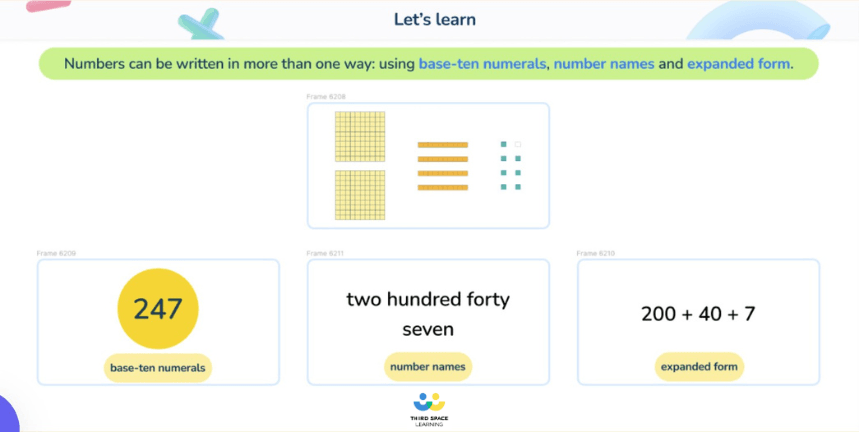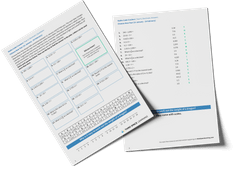Math teaching support you can trustone-on-one tutoring sessionsschools supported

[FREE] Fun Math Games & Activities Packs

Always on the lookout for fun math games and activities in the classroom? Try our ready-to-go printable packs for students to complete independently or with a partner!

# What Are Base Ten Blocks? Explained For Elementary Schools

Base Ten Blocks are a versatile addition to the elementary math classroom. As more schools adopt a math mastery approach to teaching math, interest has grown in the use of concrete resources and math manipulatives to introduce and explore math topics.

Base Ten Blocks are versatile manipulatives which allow teachers and students to represent and understand numbers. They can be used to teach a large portion of the math curriculum including place value, calculation and decimals throughout the elementary age range. In this blog, we’ll give you an introduction to Base Ten Blocks and their use in the elementary setting and provide you with practice questions and worked examples that could be answered using Base Ten Blocks.

### What are Base Ten Blocks?

Base Ten Blocks are coloured plastic or wooden blocks that are used to represent numbers. There are four different Base Ten Blocks blocks. They are usually used to represent 1000, 100, 10 and 1.

### Advantages of Base Ten Blocks blocks

• Base Ten Blocks are proportionally correct. Unlike place value counters or Cuisenaire rods, Base Ten Blocks are proportionally correct. This means ten ‘ones’ cubes are the same size as one ‘ten’ rod and this continues with each larger piece. This allows children to understand the relationship between the different columns on a place value grid.
• Base Ten Blocks can be used to assist with regrouping and exchanging in addition and subtraction. While they are commonly used to show up to four digit numbers, the proportional nature of Base Ten Blocks also allow teachers to use them to show decimal numbers and their place value. This is particularly useful when first introducing decimals in lower elementary.
• Base Ten Blocks are easy for children to use pictorially. They can quickly and neatly draw Base Ten Blocks to either help them calculate answers or to prove their work.In our one to one tutoring, we use pictorial representations of Base Ten Blocks to support students.

### How are Base Ten Blocks used in math?

Base Ten Blocks blocks can be used to enhance students’ understanding and reasoning for many different topics. They have become a staple teaching resource in many mathematics classrooms across the country to enable students to master the curriculum.

#### Base Ten Blocks for place value

It is fundamental that children understand place value to access the rest of the mathematics curriculum. Place value ideas are explored extensively throughout elementary school and are the foundation of students’ understanding of numbers as quantities and their operations.

For example, first grade students can use Base Ten Blocks blocks to represent a two-digit number and explain how many tens and ones are in a given number.

You could stretch students further by asking, ‘Do these two representations show the same number?’

In this example, a) shows 3 tens and 2 ones which is 32 and b) shows 2 tens and 12 ones which is also 32. Students may initially disagree that they are the same as there are a different number of tens, however understanding that these representations are two different ways of showing the same number will help with addition and subtraction later on.

#### Base Ten Blocks for addition

Once students are comfortable using Base Ten Blocks to show the place value of numbers and manipulate these to create different representations, they can begin to use them to calculate.

An advantage of Base Ten Blocks over resources such as number lines or counting materials is that they can be used for three- and four-digit numbers without becoming confusing or time consuming for students to count.

Base Ten Blocks can be used alongside a written calculation in the addition algorithm to show how the calculation would be written and to help students visualize the numbers that they are working with, as shown below.

As long as students understand which Base Ten Blocks represent hundreds, tens and ones, it is as easy as counting in multiples of that number.

Having learned the addition algorithm without regrouping, students can also use Base Ten Blocks to understand how to combine 10 of a smaller unit to form 1 group of the next larger unit. This is where being able to represent numbers in more than one way becomes useful.

#### Base Ten Blocks for subtraction

In a similar fashion, Base Ten Blocks blocks can be used to teach subtraction too, both as a stand alone resource to complete calculations and alongside the subtraction algorithm.

Again, once students are comfortable subtracting without regrouping and exchanging, they can then exchange larger Base Ten Blocks blocks for ten of the smaller ones in order to be able to complete subtraction calculations with regrouping and exchanging.

#### Base Ten Blocks for decimals

Base Ten Blocks can also be used to help students to visualize decimals. Until this point, we have been using Base Ten Blocks to represent the same numbers every time – whole numbers. However, since they are just within a power of ten of one another, it is possible to scale their values down to represent tenths, hundredths and thousandths.

At this stage, students will have gained familiarity using Base Ten Blocks which will help them to understand the place value of decimal numbers when this is introduced in fourth grade.

From here, teaching addition and subtraction of decimals and their relationships to fractions (and later percentages) can follow the same pattern as with whole numbers as discussed earlier.

### When do children use Base Ten Blocks in school?

In elementary school, students can use Base Ten Blocks blocks beginning in pre-K, when they are first discovering and understanding number, through to fourth and fifth grade when they are working with fractions, decimal numbers and high-level addition and subtraction. Similarly, they can be used to help children with fluency in calculation and to reason and problem solve. Children can use Base Ten Blocks blocks to prove their answers to a question, as well as represent numbers.

Aside from helping children to understand the number system and how numbers relate to one another, Base Ten Blocks can help children to develop skills for their lives outside the classroom. Most notably, they allow children to develop problem solving and reasoning skills which they can then apply to their wider lives.

### Base Ten Blocks worked examples

1. What whole number is represented?

This is completed by counting the tens and ones.

Students could then be extended by asking them to show this in a different way (maybe 7 tens and 17 ones or with a different resource, such as place value counters)

2. Complete the part-whole model using Base Ten Blocks.

This is drawn as if they were the children’s jottings to record the Base Ten Blocks.

There are multiple different answers to this problem, as long as the drawn Base Ten Blocks total 350, since there are already 15 ‘ones’ shown.

A potential misconception here will be to immediately draw 300 and 60.

3. Fill in the missing number

Again, I have completed this using both the jottings and column addition, as you may expect students to.

Emphasize that we begin on the right to ensure that if we need to exchange, we can.

4. 637 – 184

In this calculation, I have set out the column subtraction with the Base Ten Blocks visually, just as I would if I were teaching it to students with the concrete resource.

The question has provided the Base Ten Blocks here, but the question could just give the calculation and expect the children to draw the Base Ten Blocks themselves.

I began on the right as you would for the subtraction algorithm and I noticed that an exchange needed to be completed which I have shown in my working out.

5. Which decimal number is represented?

6. Third grade is fundraising for charity. Their target is $3,250. Class A raised$1,124, Class B raised $952. How much does Class C need to raise? First, I have added$1,124 and $952 using the Base Ten Blocks. This will tell me how much has already been raised. Then, I need to subtract my answer from the target –$3,250.

The answer to the question is that Class C needs to raise \$1,174.

### Base Ten Blocks practice questions

1. What number is represented? How could you represent the same number in another way?

Children could also show this with 50+13, 40+23. Encourage them to not go too much further as it becomes difficult to count. Other options include bar models, part-whole models, place value counters or number lines.

2. Complete the part-whole model using Base Ten Blocks.

Answer: Any combination which makes 4,503. Discourage children from drawing 503 ones (or similar) as this is not helpful and wastes time.

3. Find the missing number

4. 561 – 324

5. Which decimal number is represented?

6) There are 5,832 seats in a stadium.

The home team have sold 3,418 tickets and the away team have sold 833.

How many tickets are left?

#### What are Base Ten Blocks in math?

Base Ten Blocks are a versatile manipulative resource which can be used to represent numbers.

#### Are Dienes and Base Ten Blocks the same?

Yes. Dienes are the blocks that Professor Zoltan Dienes produced to help children understand the number system. We sometimes use his name for the same materials.

#### Why are Base Ten Blocks useful?

Base Ten Blocks blocks have multiple uses in every elementary classroom. All children can use them to represent numbers in their place value units, but they can also be used for addition, subtraction and decimals.

#### How do you subtract with Base Ten Blocks?

To subtract with Base Ten Blocks, first make the number that you are subtracting from (the minuend) out of Base Ten Blocks. Next, physically remove the number that you are subtracting (the subtrahend), remembering to begin on the right in case any exchanges are needed. The answer is the Base Ten Blocks that you have left.

Do you have students who need extra support in math?
Give your students more opportunities to consolidate learning and practice skills through personalized math tutoring with their own dedicated online math tutor.

Each student receives differentiated instruction designed to close their individual learning gaps, and scaffolded learning ensures every student learns at the right pace. Lessons are aligned with your state’s standards and assessments, plus you’ll receive regular reports every step of the way.

Personalized one-on-one math tutoring programs are available for:

The content in this article was originally written by primary school teacher Lauren Marlow and has since been revised and adapted for US schools by elementary and middle school teacher Kathleen Epperson.##### Lauren Marlow
Lauren is a primary school teacher, maths mastery specialist and former maths lead who teaches in schools across Leicestershire, alongside writing blogs and resources for Third Space Learning.
xx

#### [FREE] Fun Math Games & Activities Packs

Individual packs containing fun math games and activities to complete in the classroom.

The activities are designed to be fun, flexible and suitable for a range of abilities.